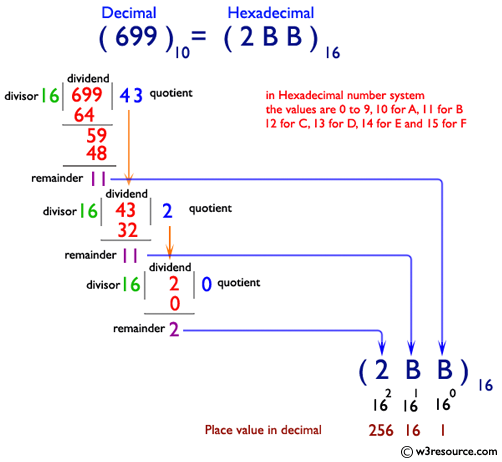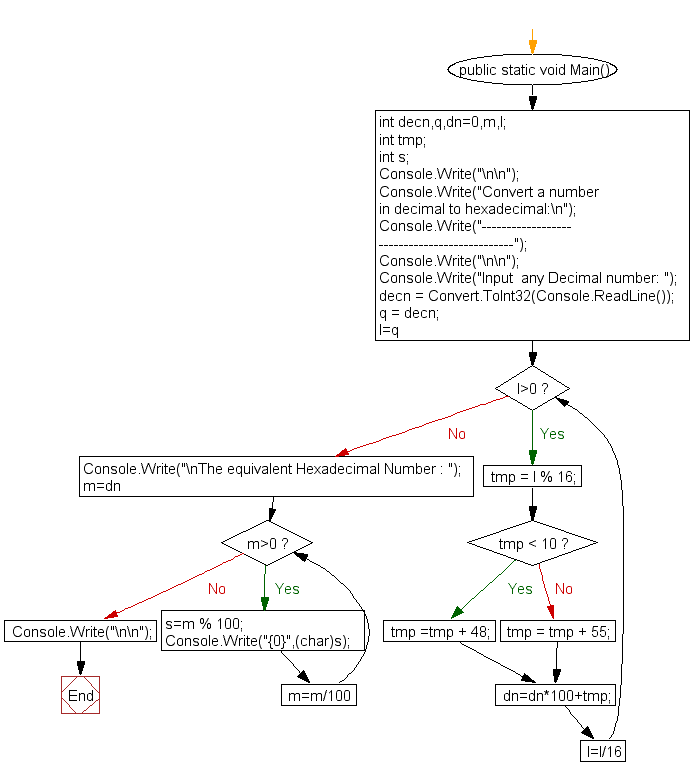﻿ C# - Convert a number in decimal to hexadecimal - w3resource# C# Sharp Exercises: Convert a number in decimal to hexadecimal

## C# Sharp For Loop: Exercise-55 with Solution

Write a program in C# Sharp to convert a decimal number to hexadecimal.Sample Solution:-

C# Sharp Code:

``````using System;
public class Exercise55
{
public static void Main()
{
int decn,q,dn=0,m,l;
int tmp;
int s;

Console.Write("\n\n");
Console.Write("Convert a number in decimal to hexadecimal:\n");
Console.Write("---------------------------------------------");
Console.Write("\n\n");

Console.Write("Input  any Decimal number: ");
q = decn;
for(l=q;l>0;l=l/16)
{
tmp = l % 16;
if( tmp < 10)
tmp =tmp + 48;
else
tmp = tmp + 55;
dn=dn*100+tmp;
}
Console.Write("\nThe equivalent Hexadecimal Number : ");
for(m=dn;m>0;m=m/100)
{
s=m % 100;
Console.Write("{0}",(char)s);
}
Console.Write("\n\n");
}
}
```
```

Sample Output:

```Convert a number in decimal to hexadecimal:
---------------------------------------------
Input  any Decimal number: 1015
The equivalent Hexadecimal Number : 3F7
```

Flowchart:C# Sharp Code Editor:

Contribute your code and comments through Disqus.

What is the difficulty level of this exercise?

Test your Programming skills with w3resource's quiz.

﻿